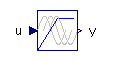Fluid Inertia - MapleSim Help

Fluid Inertia

Model the effect of fluid inertia in a pipeDescription The Fluid Inertia component models the effect of fluid inertia in a pipe, that is, the pressure differential is proportional to the rate of change of the flow rate.Equations $p={p}_{A}-{p}_{B}=\frac{L\mathrm{\rho }}{A}\frac{\mathrm{d}q}{\mathrm{d}t}$ $q={q}_{A}=-{q}_{B}$Variables

 Name Units Description Modelica ID $p$ $\mathrm{Pa}$ Pressure across component p $q$ $\frac{{m}^{3}}{s}$ Flow rate through component qConnections

 Name Description Modelica ID $\mathrm{portA}$ Upstream hydraulic port portA $\mathrm{portB}$ Downstream hydraulic port portBParameters

 Name Default Units Description Modelica ID $A$ $8·{10}^{-5}$ ${m}^{2}$ Cross-sectional area of pipe A $L$ $1$ $m$ Length of pipe L